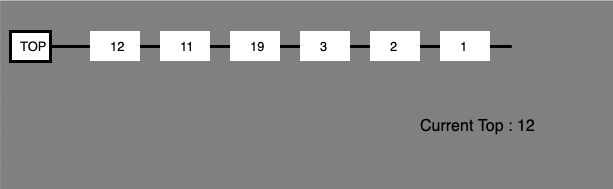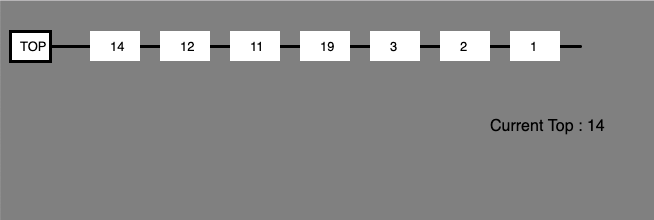# p5.js | Push Operation on Stack

• Last Updated : 04 Oct, 2021

What is Stack?
The stack is a linear data structure like an array in which only the last inserted element can be accessed. This data structure works over the principle Last In First Out (LIFO). It is very fast since it has to access only last added element so it requires constant time to do so having complexity O(1)
Stacks should be used over arrays when we need to work with data in the LIFO form. Push operation on the stack is used to adds an item in the stack. If the stack is full, then it is said to be an Overflow condition.Basic skeleton of stack: Below example run using “\$node skeleton.js” command to get basic stack skeleton.

## javascript

 `// Define Stack function ` `function` `Stack(array) {` `    ``this``.array = [];` `    ``if` `(array) ``this``.array = array;` `}`   `// Add Get Buffer property to object` `// constructor which slices the array` `Stack.prototype.getBuffer = ``function``() {` `    ``return` `this``.array.slice();` `}`   `// Add isEmpty properties to object constructor` `// which returns the length of the array` `Stack.prototype.isEmpty = ``function``() {` `    ``return` `this``.array.length == 0;` `}`   `// Instance of the stack class` `var` `stack1 = ``new` `Stack(); ``// Stack { array: [] }`   `console.log(stack1);`

Example: This example describe the push operation on stack.

## javascript

 `` `` `    `  `` `    ``Stack push operation` `    `  `    ``` `    `  `    ```   `    ``` `    `   `` `    ``<script>`   `        ``// Define Stack function ` `        ``function` `Stack(array) {` `            ``this``.array = [];` `            ``if` `(array) ``this``.array = array;` `        ``}` `        `  `        ``// Add Get Buffer property to object ` `        ``// constructor which slices the array` `        ``Stack.prototype.getBuffer = ``function``() {` `            ``return` `this``.array.slice();` `        ``}` `        `  `        ``// Add isEmpty properties to object constructor` `        ``// which returns the length of the array` `        ``Stack.prototype.isEmpty = ``function``() {` `            ``return` `this``.array.length == 0;` `        ``}` `        `  `        ``// Instance of the stack class` `        ``var` `stack1 = ``new` `Stack(); ``//Stack { array: [] }` `        `  `        ``// Add Push property to object constructor which` `        ``// push elements to the array` `        ``Stack.prototype.push = ``function``(value) {` `            ``this``.array.push(value);` `        ``}` `        `  `        ``function` `setup() {` `        `  `            ``// Create Canvas of size display width * 300` `            ``createCanvas(displayWidth, 300);` `        ``}` `        `  `        ``function` `draw() {` `        `  `            ``// Set background color` `            ``background(``"grey"``);` `            `  `            ``// Set stroke weight` `            ``strokeWeight(3);` `            `  `            ``// Set stroke color` `            ``stroke(``'black'``);` `            ``line(10, 45, 90, 45);` `            ``rect(10, 30, 40, 30);` `            ``noStroke();` `            ``text(``"TOP"``, 20, 50);` `        `  `            ``// Display stack ` `            ``for``(``var` `i = stack1[``'array'``].length-1; i >= 0; i--) {` `                ``var` `p = 10;` `                ``translate(70, 0);` `                ``strokeWeight(3);` `                ``stroke(``'black'``);` `                ``line(10+p, 45, p+80, 45);` `                ``noStroke();` `                ``rect(10+p, 30, 40+p, 30);` `                ``text(stack1[``'array'``][i], 40, 50);` `                ``p +=10;` `            ``}` `        ``}` `        `  `        ``// Call to push operation` `        ``stack1.push(1);` `        ``stack1.push(2);` `        ``stack1.push(3);` `        ``stack1.push(19);` `        ``stack1.push(11);` `        ``stack1.push(12);` `    ``</script>` `</body>`   `</html>                                    `

Output:After Pushing ’14’ by calling stack1.push(14) function to the top of stack.My Personal Notes arrow_drop_up
Recommended Articles
Page :